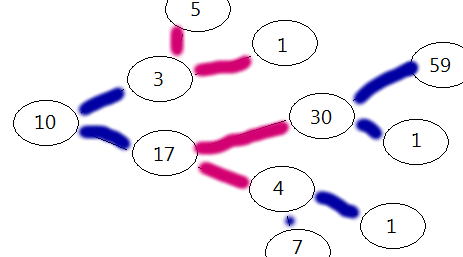# The Game of Subtracting PrimesIvan and Jake are playing a game with the following rules:

1. The game starts with a parameter N which is a positive integer.
2. Ivan always plays first.
3. In each turn, the player can either choose to add or subtract the largest prime smaller than N, to N.
4. The loser is the person who cannot continue. The other person wins by default.

Example

 1 2 3 4 5 6 N=10 Ivan: N -> N + 7 = 17 Jake: N -> N - 13 = 4 Ivan: N -> 4 - 3 = 1 Ivan wins because Jake cannot continue the game. 

Now, suppose that Ivan and Jake know how to play optimally. For how many starting values of $N < 10^4$ does Jake win?

×